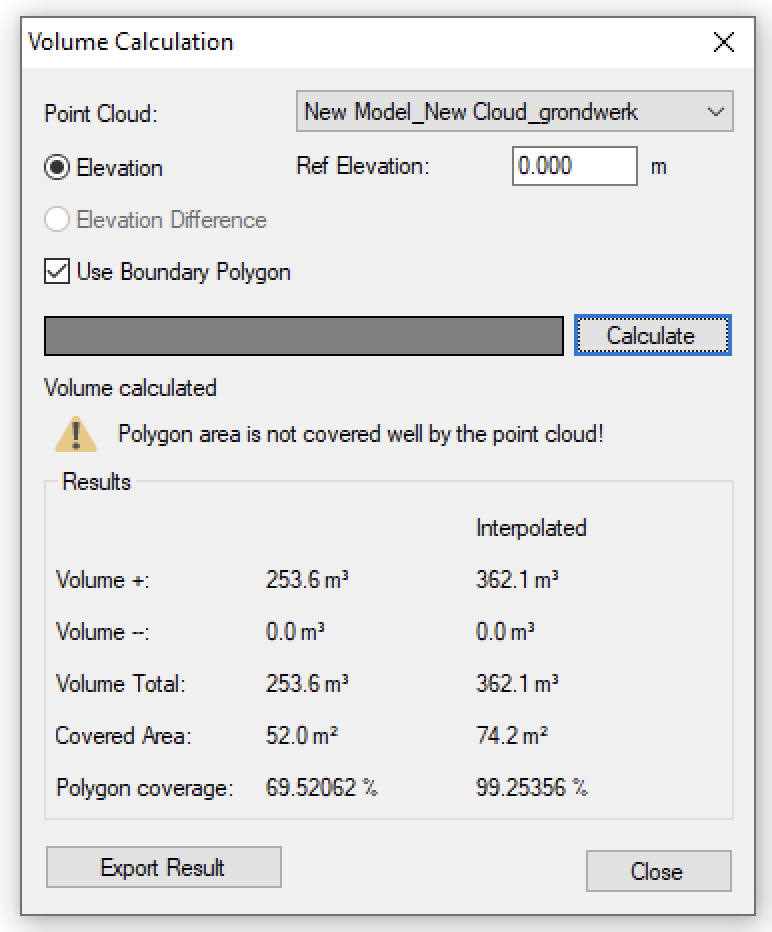Introduction:

The point cloud volume calculation has been upgraded with the option to calculate interpolated volumes.

Previous versions of Pythagoras always used the most uniform resolution to calculate volumes. This meant that sometimes, when certain parts of the point cloud did not have that same resolution, gaps avoided the complete area to be used to calculate the volume of a project.

This was visualized by a percentage in the volume calculation report.

Pythagoras will be able to calculate an interpolated volume for parts/holes of the point cloud where the resolution does not match the chosen resolution for general point cloud volume calculation.

The new report will show two values, one for the general volume calculation and one for the interpolated volume calculation.

When using a boundary polygon, the interface will show two values. One for the normal volume and one for the interpolated part. Also a percentage will be show that represents the coverage of the polygon area.

When not using a boundary polygon, the interface will not show the coverage percentages, but only the volume values.

Wanneer geen grenspolygoon gebruikt wordt kan Pythagoras geen percentages tonen voor de dekking, maar enkel een waarde voor het niet-geïnterpoleerde en het geïnterpoleerde volume.Video:

The following video shows how this works: